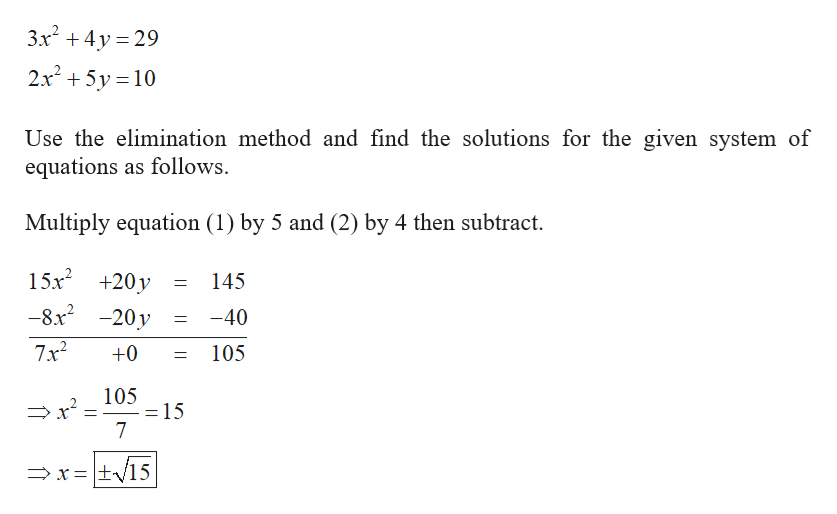# Use the elimination method to find all solutions of the system of equations.  3x2 + 4y = 292x2 + 5y = 10

Question
2 views

Use the elimination method to find all solutions of the system of equations.

 3x2 + 4y = 29 2x2 + 5y = 10
check_circle

Step 1

The given system of e...help_outlineImage Transcriptionclose3x2 4y 29 2x25y 10 Use the elimination method and find the solutions for the given system of equations as follows Multiply equation (1) by 5 and (2) by 4 then subtract 15x2 +20y 145 -8x2 -20y -40 7x2 +0 105 105 = 15 7 xtv15 fullscreen

### Want to see the full answer?

See Solution

#### Want to see this answer and more?

Solutions are written by subject experts who are available 24/7. Questions are typically answered within 1 hour.*

See Solution
*Response times may vary by subject and question.
Tagged in

### Other